# Change Nickname

Current Nickname:
Search
v2.x
v2.x

# General Graph Algorithm

### `algo(khop_all)`

Khop_all is a whole-graph algorithm that does K-hop against all nodes of the entire graph, and returns the total number of k-hop neighbors found for each node.

Obviously, this algorithm is rather an expensive operation, especially on large graphs. Khop_all will be executed as a task (asynchronous execution mode, it will be pipelined if there are other tasks before it).

Configuration items for whole graph K-hop operation:

Item Data Type Specification Description
`<depth>` int >0 A reasonable value, e.g., starting from 1 and grow incrementally
`<direction>` string 'left' or 'right' (Optional) To search paths where all edges are in the same direction along the path, either left or right, or ignore edge direction if not configured

Validity of `write_back()`:

Approach Destination
To database node property `#khop_all`
To disk file RPC port

`Ultipa Graph has optimized regular node-based K-Hop and whole graph k_hop with full parallelization, in most graphs, 1-hop takes microseconds to complete, 2-hop and deeper hops take milliseconds. However, on large graphs (having hundreds of millions of nodes/edges and many hotspots, the whole-graph k-hop can take longer time to complete. The computational complexity is astronomical, and here is the simple math: Assuming we have a graph with (# of edges / # of nodes) = 100, and doing just 1 node’s 5-hop takes: 100*100*100*100*100 = 10B in terms of complexity, if we are doing this against the entire graph’s 10M nodes, this is 10B * 10M, if doing 1 node’s 5-hop takes 100ms (0.1s), doing 10M nodes’ 5-hop takes: 0.1 * 10M = 1M seconds ~= 12 Days(& nights). The idea here is that: before you do whole-graph 5-hop (or deeper), think again!`

Example 1: Whole graph 2-hop:

``````algo(khop_all).params({ depth: 2 })
``````

### `algo(connected_component)`

Basic

A connected component (shortened as CC hereafter) is a maximal subset of interconnected nodes and edges of a graph. If one CC of a graph includes all the nodes and edges of the graph, that means the number of CCs of the graph is one, in which case the graph is also said to be connected that every pair of nodes in it have path(s) connecting them.

There are two types of CCs in terms of connection direction, WCC vs. SCC:

• WCC: Weakly Connected Component, where the edge direction is ignored when judging whether two nodes are connected.
• SCC: Strongly Connected Component, where a pair of nodes are considered connected only when the paths connecting them are bidirectional (meaning for any pair of node {u, v}, there is a path from u→v, and a path from v→u).

Connected component algorithm in Ultipa Graph counts the total number of connected components in the graph and returns which nodes belong to which CC.

Configuration item for whole connected component operation:

Item Data Type Specification Description
`<cc_type>` int 1; 2 1: WCC (weakly connected)
2: SCC (strongly connected)

Validity of `write_back()`:

Approach Destination
To database node property `#connected_component`
To disk file /

Example 1: Calculate WCC of a garph

``````algo(connected_component).params({ cc_type: 1 })
``````

Apply this uQL command to a graph with below structure: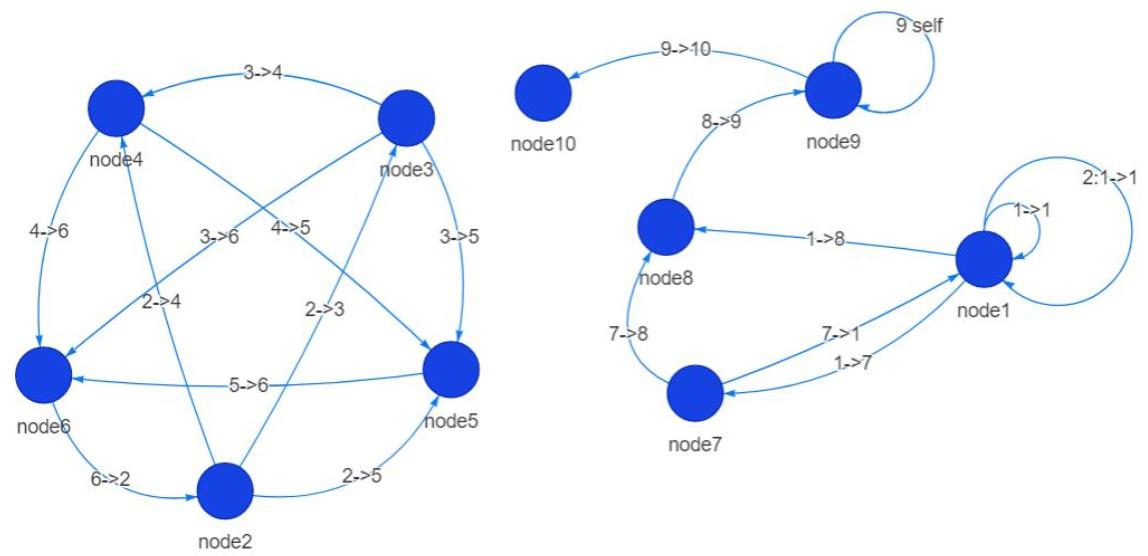Figure: A dis-connected Graph

And acquire below results: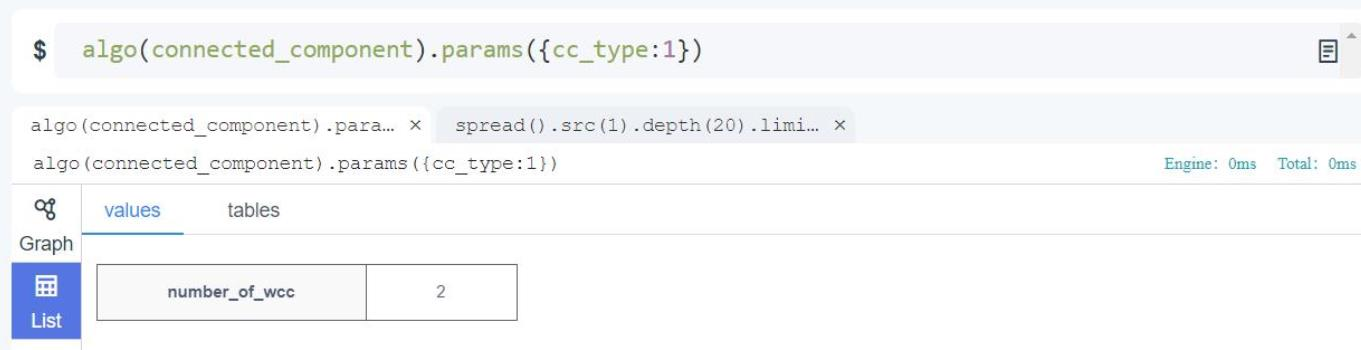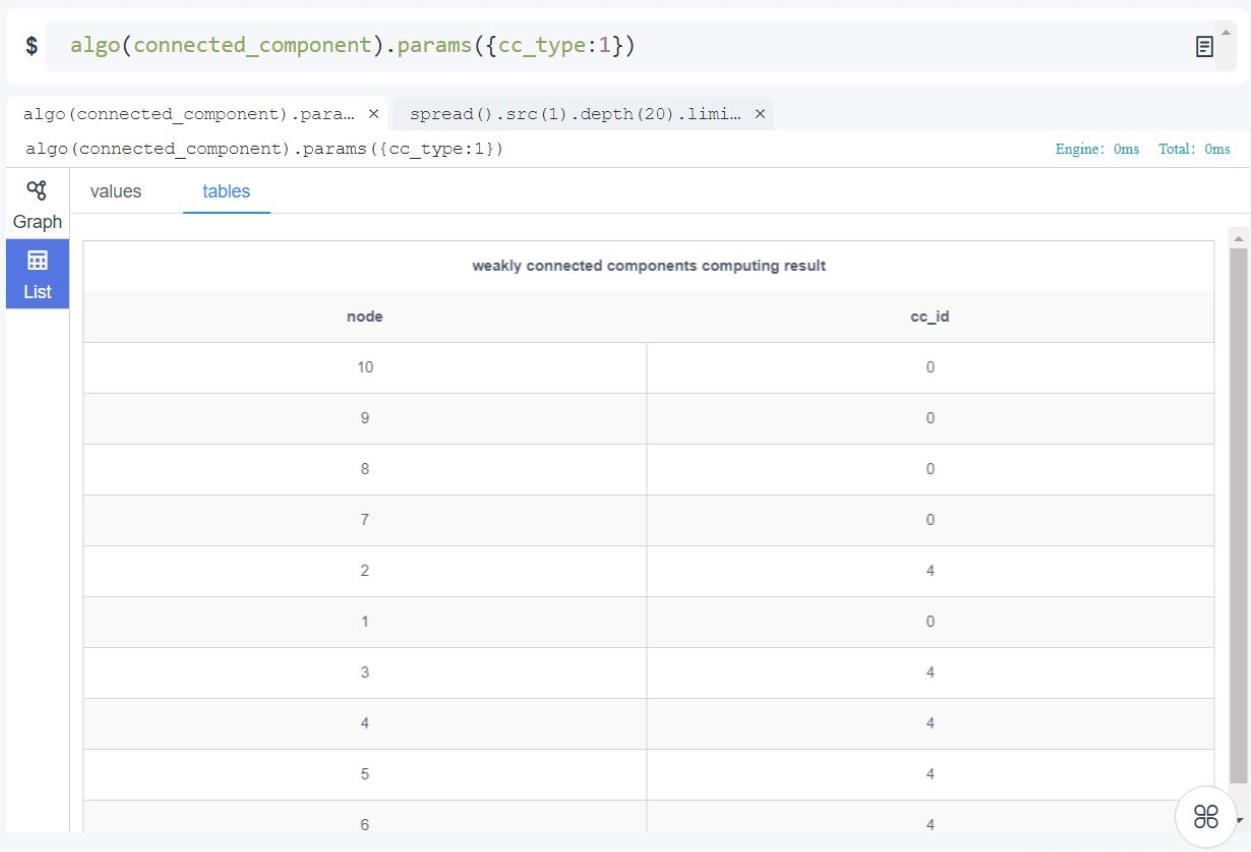Figure: Running Weakly Connected Component

### `algo(triangle_counting)`

Basic

Triangle counting algorithm counts how many triangles are there in the graphset and returns the nodes or edges forming each trangle.

Triangle counting is an algorithm that's used to determine and gauge the stability of a graph, and is often used to construct more sophisticated graph algorithms. It's widely applied to measure the cohesiveness of communities within social networks, to measure how closely connected are the accounts (account holders) to each other in a banking system, etc.

Configurations item for triangle counting operation:

Item Data Type Specification Description
`<type>` int 1; 2 1: Count by edge
2: Count by node
`<limit>` int >0; -1 The maximum number of triangles to return; -1: only return the total count of triangles without listing their nodes/edges

Validity of `write_back()`:

Approach Destination
To database /
To disk file RPC port

It’s imperative to understand the difference between the `<type>` values:

• Count by edge: Each triangle is defined by its edges.
• Count by node: Each triangle is defined by its nodes.

Obviously, a triangle defined by nodes will be counted as different triangles when being defined by edges if there are multiple edges between any two of its nodes. In other words, 'count by node' will churn out a number smaller than 'count by edge'.

To best illustrate this, check out the below screenshot: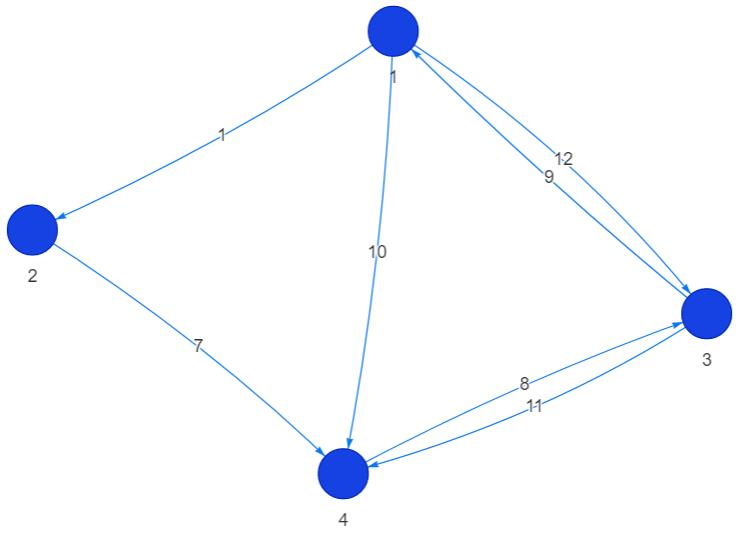Figure: Triangle Counting Illustrated

When aggregating by nodes, there are only 2 triangles; on the other hand, there are 5 triangles if we aggregate by edges (1 + 122).

It’s worthwhile to point out that some graph databases can NOT count and aggregate triangles by edges, which just misses the point of a graph database. Mathematically, a unique triangle is determined by 3 circularly connected edges sharing endpoints with each other.

Example: Launch Triangle Counting by edge against the graph in the previous illustration

``````algo(triangle_counting).params({type: 1, limit: 20}).write_back()
``````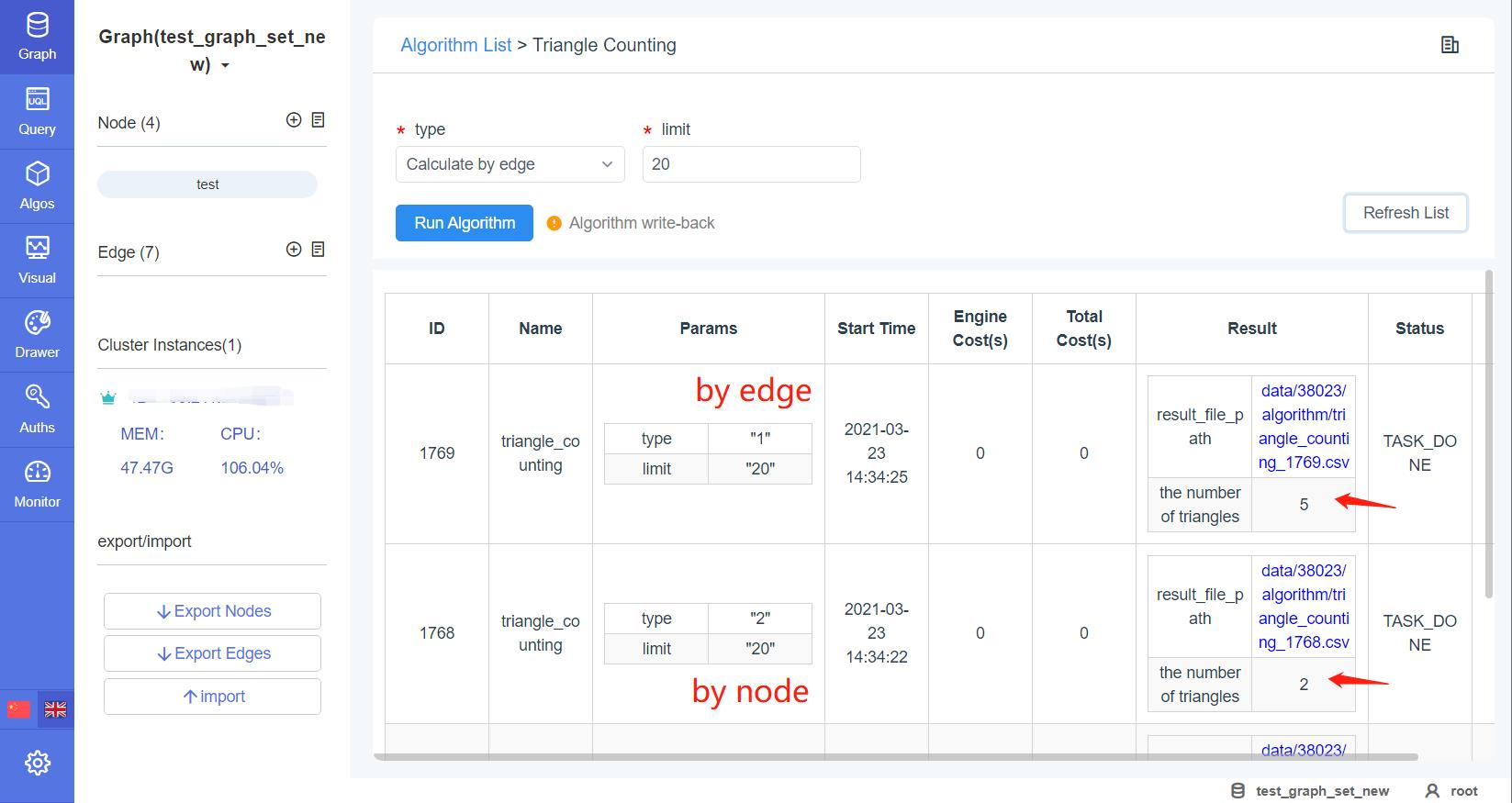Figure: The 2 Modes of Triangle Counting (Note the Difference)

### `algo(common_neighbours)`

Real-time

Common Neighbors calculates the shared (common) neighbors (1-hop) between two nodes, returns both the total number and the neighbors list.

Configuration items for Common Neighbors operation:

Item Data Type Specification Description
`<node_id1>` int Ultipa ID /
`<node_id2>` int Ultipa ID /

Validity of `write_back()`:

Not supported.

Example: Calculate the common neighbors of node 12 and 13

``````algo(common_neighbours).params({node_id1: 12, node_id2: 13})
``````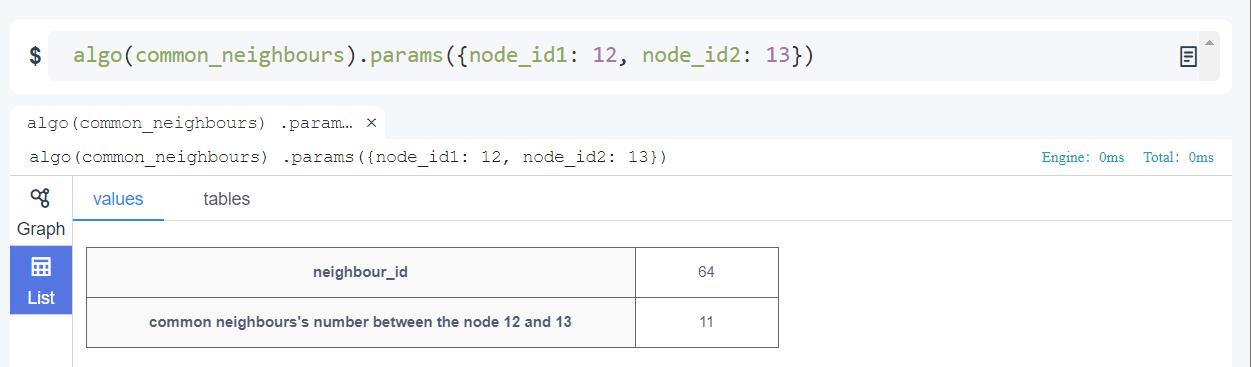Figure: Calculating Common Neighbors

To validate the accuracy of Common Neighbors, use template query to the rescue - calculate 2-hop paths from node 12 to 13 and use `\$count_distinct` to count the distinctive in-between nodes:

``````t(p).n(12).e().n(a).e().n(13).limit(10000).return({\$count_distinct: a})
``````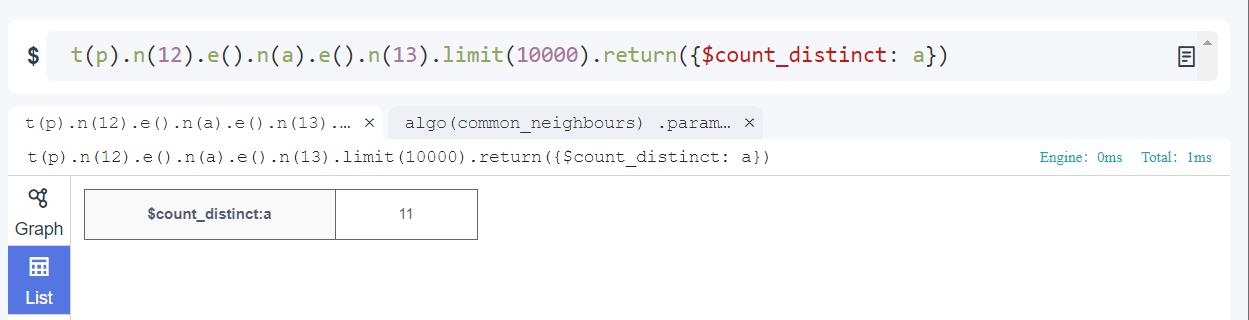Figure: Using Template Query to Validate Common Neighbors

### `algo(subgraph)`

Real-time

Subgraph algorithm calculates the induced subgraph based on the given subset of nodes, by returning all the edges whose endpoints both belong to the given subset of nodes.

Configuration item for Subgraph operation:

Item Data Type Specification Description
`<node_ids>` []int Ultipa ID The subset of nodes

Validity of `write_back()`:

Approach Destination
To database /
To disk file RPC port

Example: Calculate the induced subgraph using nodes [1,7,8,9]

``````algo(subgraph).params({node_ids: [1,7,8,9]})
``````

Run this example in uQL CLI: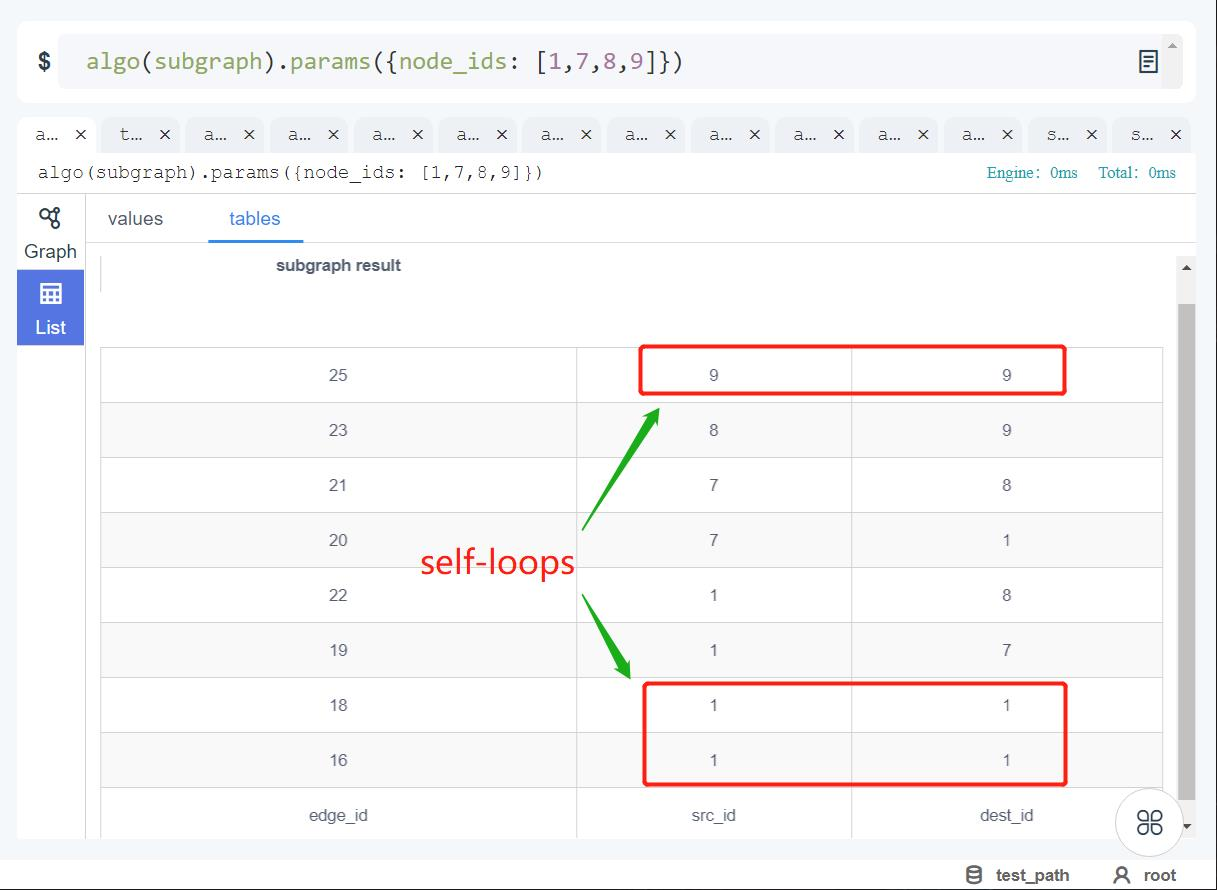Figure: Running Subgraph Algorithm in CLI

The screenshot below shows how subgraph algorithm is executed with Algos module in Ultipa Manager: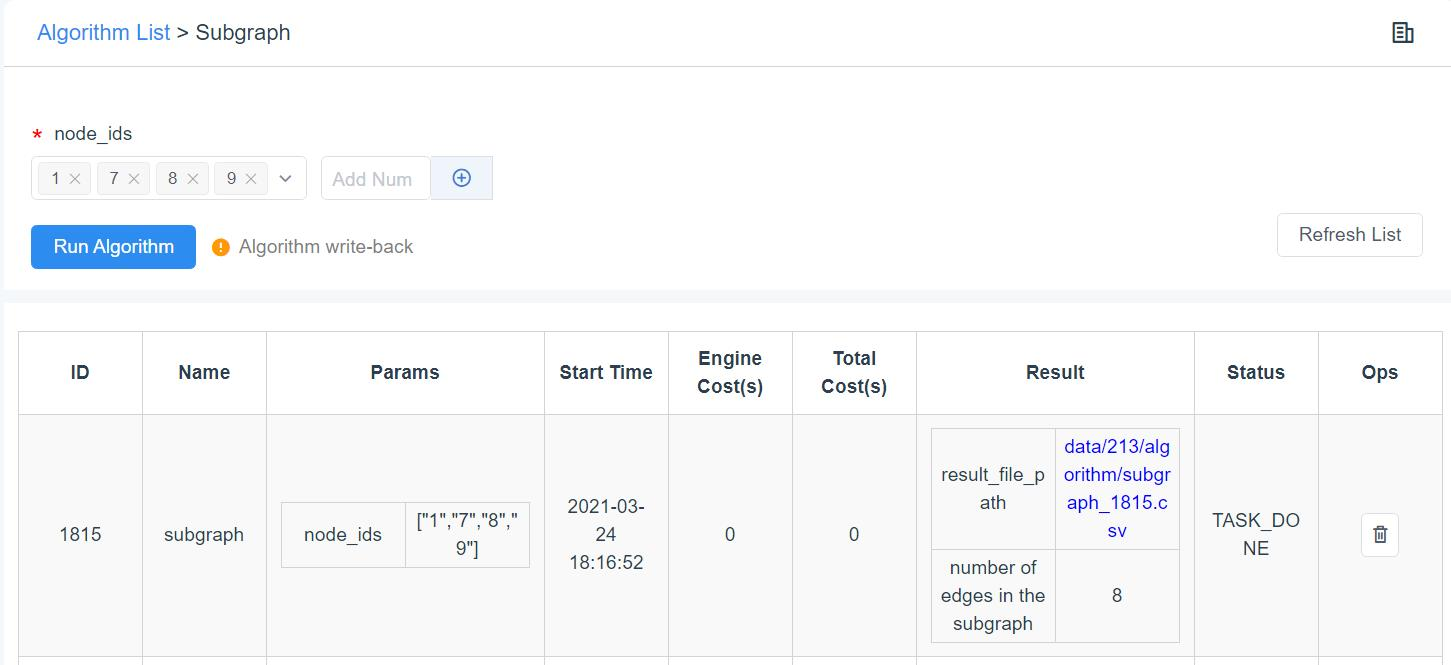Figure: Running Subgraph Algorithm with Algos

Subgraph algorithm by definition is equivalent to running a 1-hop `autoNet()` query with the given nodes as sources. However, exception occurs when there are self-loops (edges starting and pointing to the same nodes) on the given nodes, and these self-loops will not be calculated by the 1-hop `autoNet()` query. The reason is that `autoNet()` does not calculate cycles, which are the paths whose source and destination are identical. Compare the difference by running `autoNet()` query in Ultipa Manager:

``````autoNet().srcs(1,7,8,9).depth(1).limit(100).select(*)
``````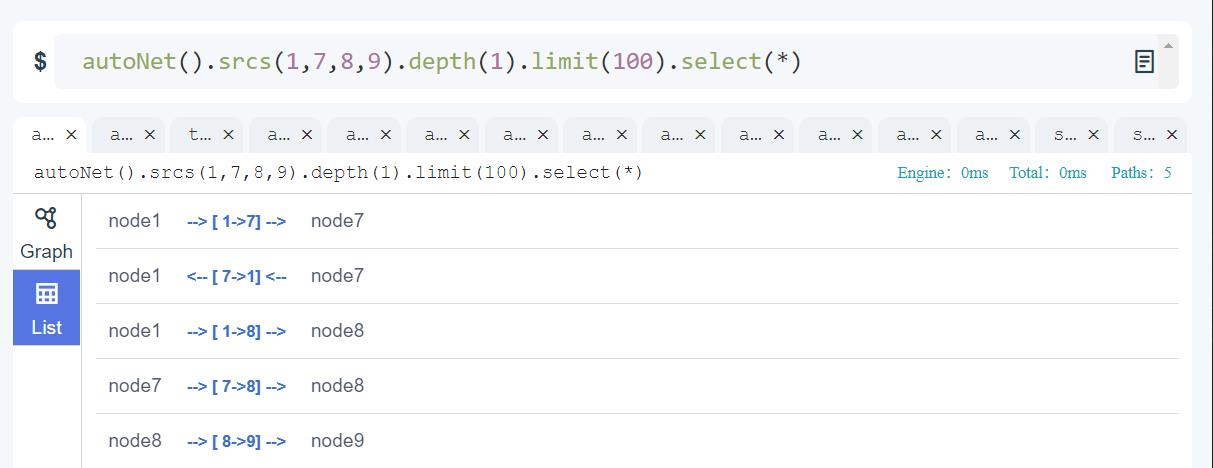Using autoNet() to Validate Subgraph Algorithm Calculation Correctness